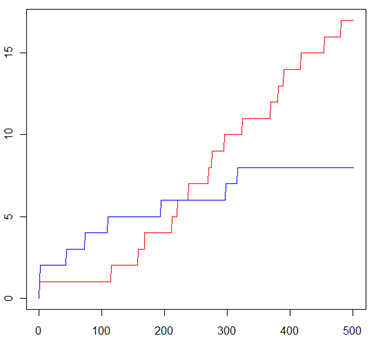## Friday, February 19, 2010

### An Anonymity computation using R

Back in August 2008 I posted on some research I did on traffic confirmation attacks. Traffic analysis is a collection of techniques for inferring communication relationships without having access to the content that is being communicated. Traffic confirmation is a special sub-case that attempts to infer communication relationships amongst users whose communications are mediated through an anonymity system.

The graph below shows two curves representing the observed frequency of recipients from a MIX anonymity system. The attacker is targeting a particular user Alice, and the red curve represents the minimal number of times her communication partners are observed, while the blue curve represents the maximal number of observations for all other recipients. The graph is created from an anonymity system with N = 20,000 users, using a MIX of batch size 50 and assuming Alice has 20 communication partners.The graph shows that after the attacker has observed about 250 messages from Alice, each of her recipients has been observed more often than any of the other recipients. Therefore an attacker can conclude that the 20 most frequently observed recipients are the recipients of Alice, and the anonymity of her partners has been broken. The details are explained in the previous post.

What surprised me was how easily the data for this graph could be programmed in the R programming language. The native vector operations of R makes this all very simple, and the code is below.

b = 50
N = 20000
m = 20
Nset = c(1:N)
mset = c(1:m)
N.plot = 0
m.plot = 0
m.min = 0
N.max = 1
s = sample(N, b, replace=T)
s = c(s, sample(mset,1,replace=T))
for ( t in c(1:500) )
{
s = c(s, sample(Nset, b, replace=T), sample(mset,1,replace=T))
ts = table(s)
m.min = min(ts[c(1:m)])
N.max = max(ts[-c(1:m)])
N.plot = c(N.plot, N.max)
m.plot  = c(m.plot, m.min)
}
plot(m.plot, type="l", col = "red")
lines(N.plot, col = "blue")